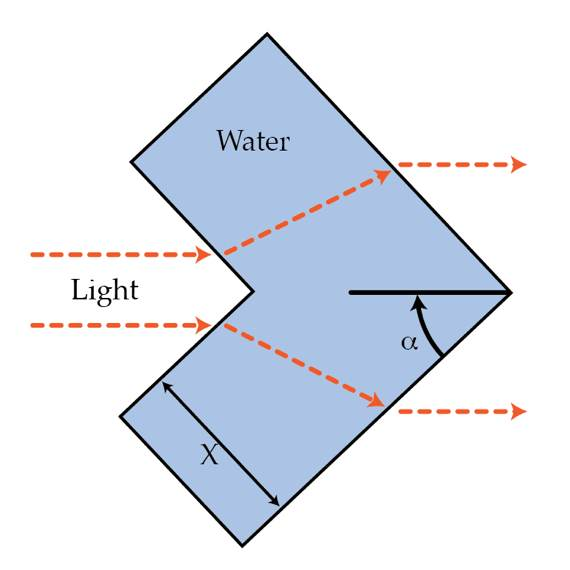# Hiding behind a goldfish

Things can be made invisible in one direction using a combination of lenses and mirrors which would bend the light around them. One way to hide a certain area is to build a big aquarium with water as shown in the picture that will bend the light.

What should be the width X of the aquarium in m so that we can hide $1~\mbox{m}$ of space? $1~\mbox{m}$ of space means the distance between the outgoing light rays in the figure is 1 m.Details and assumptions

• The refractive index of water is $1.33$.
• The prism angle $\alpha$ of the aquarium is $30^\circ$.
×